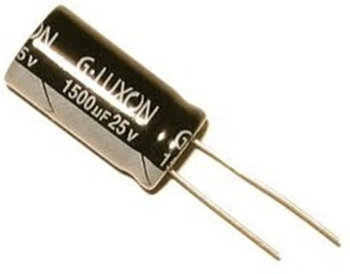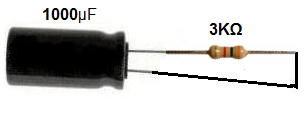﻿ How Long Does It Take to Discharge a Capacitor?# How Long Does It Take to Discharge a Capacitor?A fully charged capacitor discharges to 63% of its voltage after one time period. After 5 time periods, a capacitor discharges up to near 0% of all the voltage that it once had. Therefore, it is safe to say that the time it takes for a capacitor to discharge is 5 time constants.

To calculate the time constant of a capacitor, the formula is τ=RC. This value yields the time (in seconds) that it takes a capacitor to discharge to 63% of the voltage that is charging it up. After 5 time constants, the capacitor will discharge to almost 0% of all its voltage.

Therefore, the formula to calculate how long it takes a capacitor to discharge to is:

Time for a Capacitor to Discharge= 5RC

After 5 time constants, for all extensive purposes, the capacitor will be discharged of nearly all its voltage. A capacitor never discharges fully to zero volts, but does get very close.

### Example

Below we have a circuit of a 1000µF capacitor discharging through a 3KΩ resistor. The capacitor, at full charge, held 9 volts:One time constant, τ=RC=(3KΩ)(1000µF)=3 seconds.5x3=15 seconds. So it takes the capacitor 15 seconds to discharge up to 0 volts.